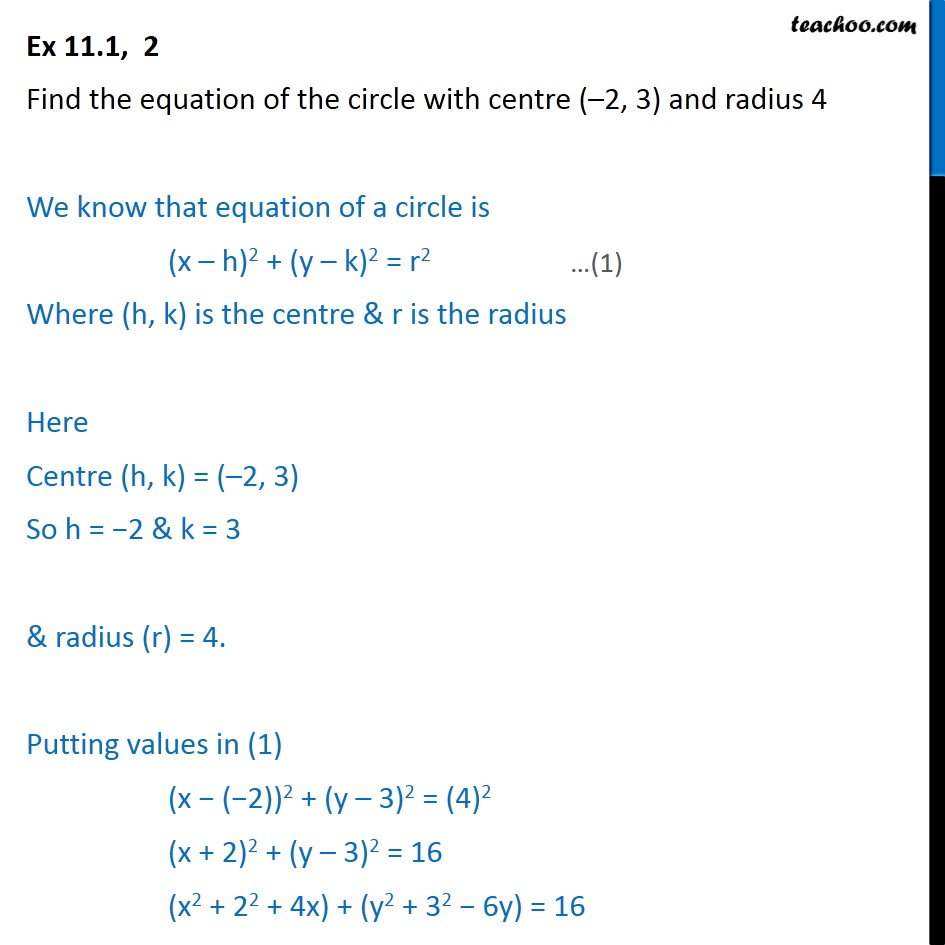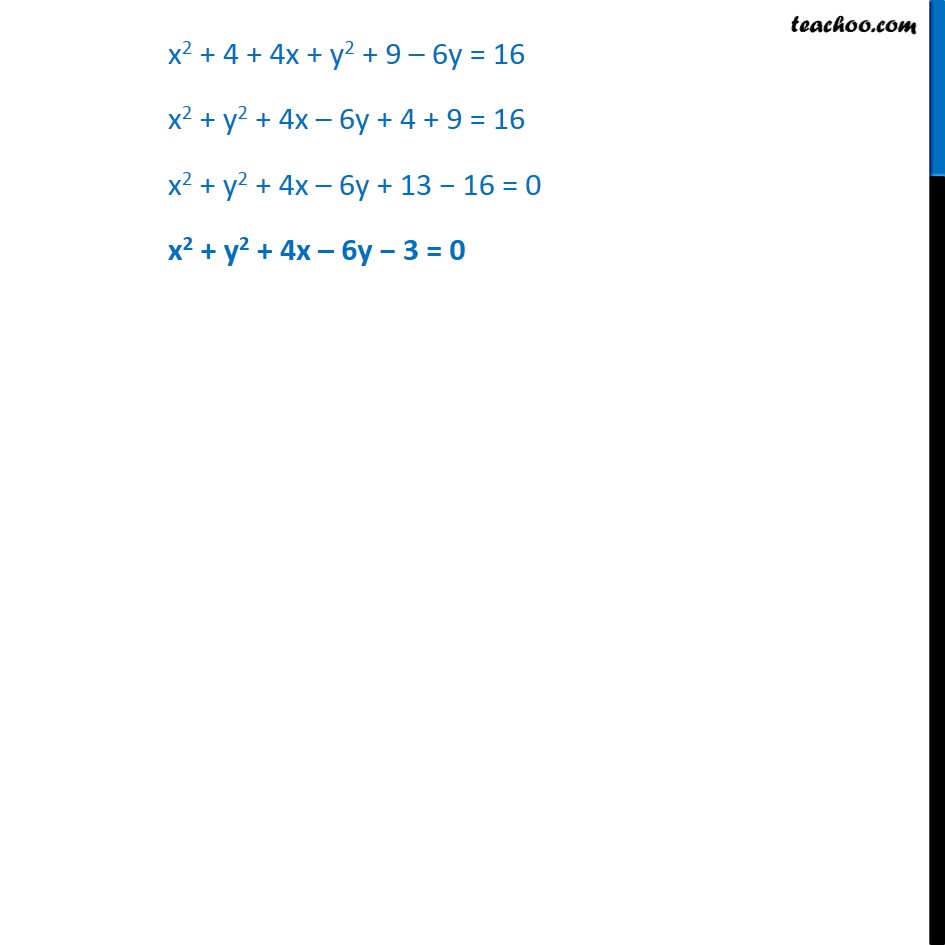Circle

Chapter 11 Class 11 Conic Sections
Concept wiseIntroducing your new favourite teacher - Teachoo Black, at only ₹83 per month

### Transcript

Ex 11.1, 2 Find the equation of the circle with centre ( 2, 3) and radius 4 We know that equation of a circle is (x h)2 + (y k)2 = r2 Where (h, k) is the centre & r is the radius Here Centre (h, k) = ( 2, 3) So h = 2 & k = 3 & radius (r) = 4. Putting values in (1) (x ( 2))2 + (y 3)2 = (4)2 (x + 2)2 + (y 3)2 = 16 (x2 + 22 + 4x) + (y2 + 32 6y) = 16 x2 + 4 + 4x + y2 + 9 6y = 16 x2 + y2 + 4x 6y + 4 + 9 = 16 x2 + y2 + 4x 6y + 13 16 = 0 x2 + y2 + 4x 6y 3 = 0